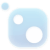# Top 20 NuGet blas Packages## F# Tensor (multidimensional array) library with SIMD and GPU acceleration

Tensor (n-dimensional array) library for F# Core features: - n-dimensional arrays (tensors) in host memory or on CUDA GPUs - element-wise operations (addition, multiplication, absolute value, etc.) - basic linear algebra operations (dot product, SVD decomposition, mat...
Intel® Math Kernel Library (Intel® MKL) is a computing math library of highly optimized, extensively threaded routines for applications that require maximum performance. This package provides C programming language interfaces. Intel MKL C language interfaces can be called from applications written i...
Intel® Math Kernel Library (Intel® MKL) is a computing math library of highly optimized, extensively threaded routines for applications that require maximum performance. This package provides C programming language interfaces. Intel MKL C language interfaces can be called from applications written i...
Intel® Math Kernel Library (Intel® MKL) is a computing math library of highly optimized, extensively threaded routines for applications that require maximum performance. This package provides C programming language interfaces. Intel MKL C language interfaces can be called from applications written i...
Intel® Math Kernel Library (Intel® MKL) is a computing math library of highly optimized, extensively threaded routines for applications that require maximum performance. This package provides C programming language interfaces. Intel MKL C language interfaces can be called from applications written i...
Intel® Math Kernel Library (Intel® MKL) is a computing math library of highly optimized, extensively threaded routines for applications that require maximum performance. This package provides C programming language interfaces. Intel MKL C language interfaces can be called from applications written i...
Intel® Math Kernel Library (Intel® MKL) is a computing math library of highly optimized, extensively threaded routines for applications that require maximum performance. This package provides C programming language interfaces. Intel MKL C language interfaces can be called from applications written i...
Intel® Math Kernel Library (Intel® MKL) is a computing math library of highly optimized, extensively threaded routines for applications that require maximum performance. This package provides C programming language interfaces. Intel MKL C language interfaces can be called from applications written i...
Intel® Math Kernel Library (Intel® MKL) is a computing math library of highly optimized, extensively threaded routines for applications that require maximum performance. This package provides C programming language interfaces. Intel MKL C language interfaces can be called from applications written i...
Intel® Math Kernel Library (Intel® MKL) is a computing math library of highly optimized, extensively threaded routines for applications that require maximum performance. This package provides C programming language interfaces. Intel MKL C language interfaces can be called from applications written i...
Intel® Math Kernel Library (Intel® MKL) is a computing math library of highly optimized, extensively threaded routines for applications that require maximum performance. This package provides C programming language interfaces. Intel MKL C language interfaces can be called from applications written i...
Intel Math Kernel Library (Intel MKL) is a computing math library of highly optimized, extensively threaded routines for applications that require maximum performance. This package provides C programming language interfaces. Intel MKL C language interfaces can be called from applications written in ...
Intel® Math Kernel Library (Intel® MKL) is a computing math library of highly optimized, extensively threaded routines for applications that require maximum performance. This package provides C programming language interfaces. Intel MKL C language interfaces can be called from applications written i...
Intel Math Kernel Library (Intel MKL) is a computing math library of highly optimized, extensively threaded routines for applications that require maximum performance. This package provides C programming language interfaces. Intel MKL C language interfaces can be called from applications written in ...
Intel® Math Kernel Library (Intel® MKL) is a computing math library of highly optimized, extensively threaded routines for applications that require maximum performance. This package provides C programming language interfaces. Intel MKL C language interfaces can be called from applications written i...
Intel Math Kernel Library (Intel MKL) is a computing math library of highly optimized, extensively threaded routines for applications that require maximum performance. This package provides C programming language interfaces. Intel MKL C language interfaces can be called from applications written in ...
Intel® Math Kernel Library (Intel® MKL) is a computing math library of highly optimized, extensively threaded routines for applications that require maximum performance. This package provides C programming language interfaces. Intel MKL C language interfaces can be called from applications written i...
Intel® Math Kernel Library (Intel® MKL) is a computing math library of highly optimized, extensively threaded routines for applications that require maximum performance. This package provides C programming language interfaces. Intel MKL C language interfaces can be called from applications written i...
Intel Math Kernel Library (Intel MKL) is a computing math library of highly optimized, extensively threaded routines for applications that require maximum performance. This package provides C programming language interfaces. Intel MKL C language interfaces can be called from applications written in ...
Intel® Math Kernel Library (Intel® MKL) is a computing math library of highly optimized, extensively threaded routines for applications that require maximum performance. This package provides C programming language interfaces. Intel MKL C language interfaces can be called from applications written i...